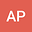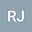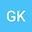The incomplete exponential \boldmath{$_pR_q(\alpha,\beta;z)$} function with some applications
•••• +1
• Ankit Pal,
• R. Jana,
• Ghazi Khammash,
• AJAY SHUKLA
Ankit Pal
Sardar Vallabhbhai National Institute of Technology

Corresponding Author:[email protected]

Author ProfileR. Jana
Sardar Vallabhbhai National Institute of Technology
Author ProfileGhazi Khammash
Al Aqsa University
Author ProfileAJAY SHUKLA
Sardar Vallabhbhai National Institute of Technology
Author Profile## Abstract

In this paper, we are motivated to establish a generalization of $_pR_q(\alpha,\beta;z)$ function in terms of incomplete exponential functions and obtain some properties of the incomplete exponential $_pR_q(\alpha,\beta;z)$ function. Further we give generating relations for incomplete exponential $_pR_q(\alpha,\beta;z)$ function. Some applications related to ground water pumping (Hydrology) modelling and probability theory have also been discussed.